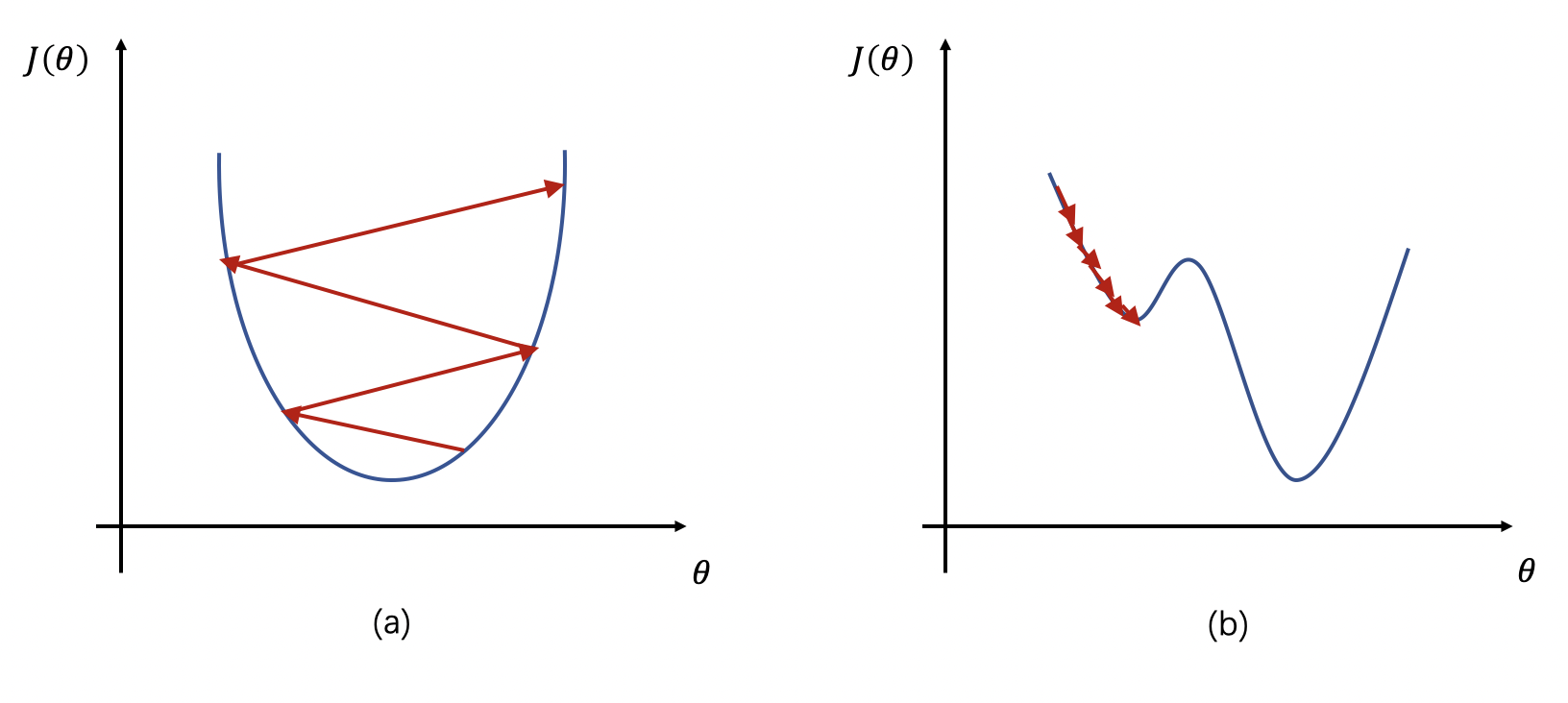## 2. 学习率对网络的影响## 3. 学习率的设置

### 3.1. 分段常数衰减（Piecewise Decay）

boundaries = [100, 200]  # 指定学习率改变的边界点为100和200
values = [1.0, 0.5, 0.1] # 指定不同区间下的学习率大小

learning_rate = 1.0  if epoch < 100
learning_rate = 0.5  if 100 <= epoch < 200
learning_rate = 0.1  if epoch >= 200

### 3.2. 指数衰减（Exponential Decay）

$$new\_learning\_rate = last\_learning\_rate * gamma$$

### 3.3. 自然指数衰减 （Natural Exponential Decay）

$$new\_learning\_rate = learning\_rate * e^{-gamma*epoch}$$

### 3.4. 多项式衰减（Polynomial Decay）

\begin{align} decay\_steps &= decay\_steps * math.ceil(\frac{epoch}{decay\_steps}) \\ new\_learning\_rate &= (learning\_rate - end\_lr) * (1 - \frac{epoch}{decay\_steps})^{power} + end\_lr \end{align}

\begin{align} epoch &= min(epoch, decay\_steps) \\ new\_learning\_rate &= (learning\_rate - end\_lr) * (1 - \frac{epoch}{decay\_steps})^{power} + end\_lr \end{align}

### 3.5. 间隔衰减 （Step Decay）

learning_rate = 0.5 # 学习率初始值
step_size = 30      # 每训练30个epoch进行一次衰减
gamma = 0.1         # 衰减率

learning_rate = 0.5    if epoch < 30
learning_rate = 0.05   if 30 <= epoch < 60
learning_rate = 0.005  if 60 <= epoch < 90
...

### 3.6. 多间隔衰减（Multi Step Decay）

learning_rate = 0.5    # 学习率初始值
milestones = [30, 50]  # 指定轮数间隔
gamma = 0.1            # 衰减率

learning_rate = 0.5    if epoch < 30
learning_rate = 0.05   if 30 <= epoch < 50
learning_rate = 0.005  if 50 <= epoch
...

### 3.7. 逆时间衰减（Inverse Time Decay）

$$new\_learning\_rate = \frac{learning\_rate}{1 + gamma * epoch}$$

### 3.8. Lambda衰减（Lambda Decay）

learning_rate = 0.5                      # 学习率初始值
lr_lambda = lambda epoch: 0.95 ** epoch  # 定义lambda函数

learning_rate = 0.5                      # 当epoch = 0时，0.5 * 0.95 ** 0 = 0.5
learning_rate = 0.475                    # 当epoch = 1时，0.5 * 0.95 ** 1 = 0.475
learning_rate = 0.45125                  # 当epoch = 2时，0.5 * 0.95 ** 2 = 0.45125
...

### 3.9. 余弦衰减（Cosine Annealing Decay）

\begin{align} \eta_t = \eta_{min} + \frac{1}{2}(\eta_{max} - \eta_{min})(1 + cos(\frac{T_{cur}}{T_{max}}\pi)), \quad T_{cur} \neq (2k+1)T_{max} \\ \eta_{t+1} = \eta_{t} + \frac{1}{2}(\eta_{max} - \eta_{min})(1 - cos(\frac{1}{T_{max}}\pi)), \quad T_{cur} = (2k + 1)T_{max} \end{align}

### 3.10. 诺姆衰减（Noam Decay）

$$new\_learning\_rate = learning\_rate * d_{mode}^{-0.5}*min(epoch^{-0.5}, epoch*warmup\_steps^{-1.5})$$

### 3.12. 线性学习率热身（Linear Warm Up）

$$lr = start\_lr + (end\_lr - start\_lr) * \frac{epoch}{warmup\_steps}$$

$$lr = learning\_rate$$

Last modification：September 18th, 2021 at 10:28 am